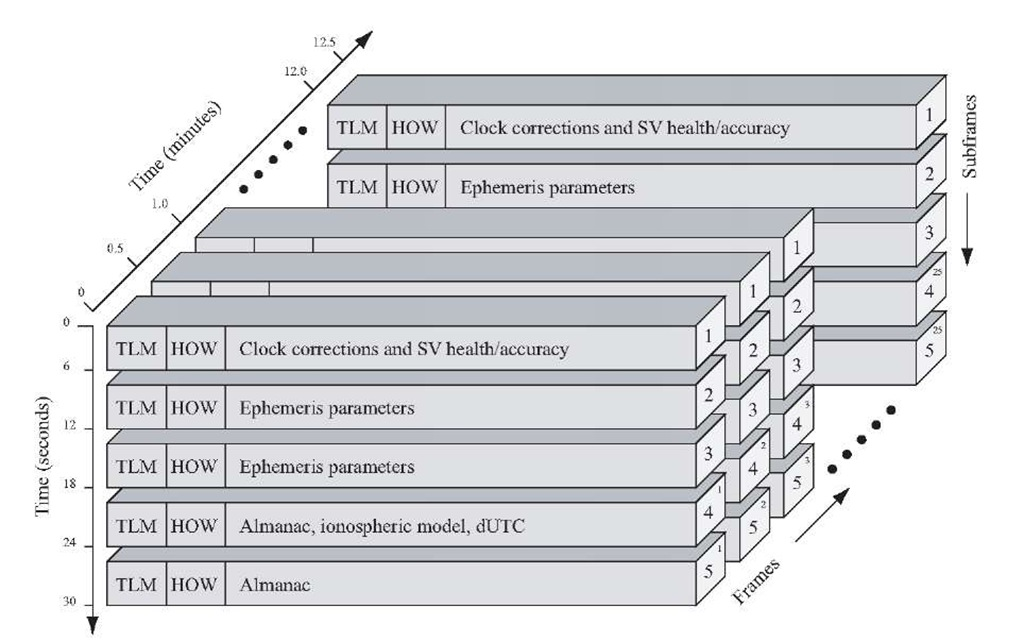# GPS导航电文格式

## 导航电文的结构### 第一数据块

• 星期数，每周星期数值+1，最大值为1023，若超过1023就会回零。
• 用户测距进度精度（URA），是对所有由GPS地面监控部分和空间星座部分引起的误差的一个统计值，0-15变化，URA数值越大，则表示从这颗卫星得到的GPS距离测量值精度越低。
• 卫星健康状况：共6比特，最高位0表示导航电文正确，1表示导航电文存在错误，后5位表示不同的错误状态。
• 时钟校正参数：用来修正卫星时钟的钟差。
• 群波延时校正值：单频接收机利用该值校正群波延迟。
• 时钟数据期号：用10比特数据表示时钟数据块的“期号”，告诉接收机在时钟校正数据更新时才去处理数据中的时钟校正参数。

### 第二数据块

1 $t_{oe}$ 星历参考时间
2 $\sqrt a_s$ 卫星轨道长半轴$a_s$的平方根
3 $e_i$ 轨道偏心率
4 $i_0$ $t_{oe}$时的轨道倾角
5 $Ω_0$ 周内时等于0时的轨道升交点赤经
6 $\omega$ 轨道近地角距
7 $Ｍ_0$ $t_{oe}$时的平近点角
8 $\Delta n$ 平均运动角速度校正值
9 $\stackrel{.}{i}$ 轨道倾角对时间的变化率
10 $\stackrel{.}{Ω}$ 轨道升交点赤经对时间的变化率
11 $C_{uc}$ 升交点角距余弦调和校正振幅
12 $C_{us}$ 升交点角距正弦调和校正振幅
13 $C_{rc}$ 轨道半径余弦调和校正振幅
14 $C_{rs}$ 轨道半径正弦调和校正振幅
15 $C_{ic}$ 轨道倾角余弦调和校正振幅
16 $C_{is}$ 轨道倾角正弦调和校正振幅

### 第三数据块

• 第4子帧的2,3,4,5,7,8,9,10页提供PRN号为25-32号卫星的历书。
• 第4子帧的第18页提供电离层校正参数以及GPS时间UTC之间关系的参数。
• 第4子帧第25页提供PRN号为25-32号卫星的卫星健康状况。
• 第5子帧1-24页提供PRN号为1-24号卫星的历书。
• 25页提供PRN号为1-24号卫星的健康状况，历书参考时间和历书参考星期数。

GPS卫星历书参数如下表：

1 $t_{oa}$ 历书参考时间
2 $\sqrt a_s$ 卫星轨道长半轴$a_s$的平方根
3 $e_s$ 轨道偏心率
4 $\delta {_i}$ 相对于0.3$\phi$的轨道倾角
5 $Ω_0$ 周内时等于0时的轨道升交点赤经
6 $\omega$ 轨道近地角距
7 $Ｍ_0$ $t_{oa}$时的平近点角
8 $\stackrel{.}{Ω}$ 轨道升交点赤经对时间的变化率
9 $a_{f0}$ 卫星时钟校正参数
10 $a_{f1}$ 卫星时钟校正参数

GPS原理与接收机设计-谢钢著